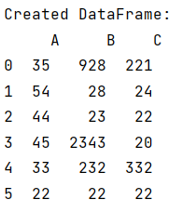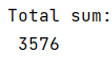# How to get total of Pandas column?

Given a Pandas DataFrame, we have to get the total of columns. By Pranit Sharma Last updated : September 22, 2023

Pandas is a special tool that allows us to perform complex manipulations of data effectively and efficiently. Inside pandas, we mostly deal with a dataset in the form of DataFrame. DataFrames are 2-dimensional data structures in pandas. DataFrames consist of rows, columns, and the data. The Data inside the DataFrame can be of any type.

## Problem statement

Given a Pandas DataFrame, we have to get the total of columns.

## Getting total of Pandas column

Pandas allows us to get the total sum of a column, but the column values should be a number. However a particular column may consist of similar values, so if we want to get the sum of unique values we can group by the values of that column and use the sum() method or if we want the sum of all the values then we can directly use the sum() method.

Note

To work with pandas, we need to import pandas package first, below is the syntax:

```import pandas as pd
```

Let us understand with the help of an example,

## Python program to get total of Pandas column

```# Importing pandas package
import pandas as pd

# Importing numpy package
import numpy as np

# Creating a dictionary
d = {
"A": [35, 54, 44, 45, 33, 22],
"B": [928, 28, 23, 2343, 232, 22],
"C": [221, 24, 22, 20, 332, 22],
}

# Creating a DataFrame
df = pd.DataFrame(d)

# Display DataFrame
print("Created DataFrame:\n", df, "\n")

# Get the sum of column B
Total = df["B"].sum()

# Display total
print("Total sum:\n", Total)
```

### Output

The output of the above program is:After, getting the sum of the column 'B',